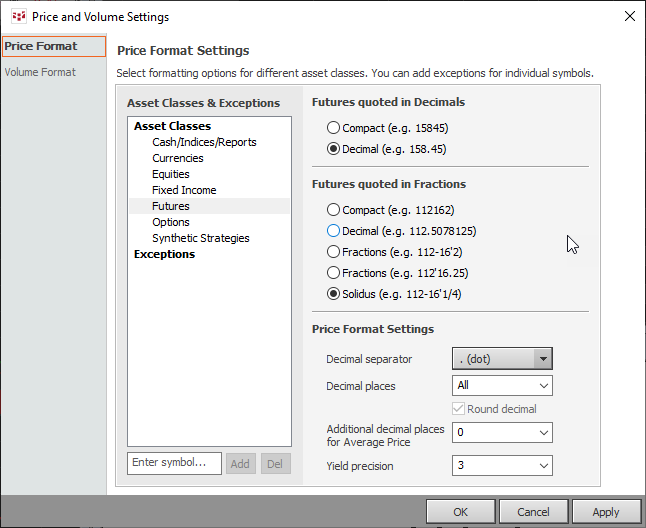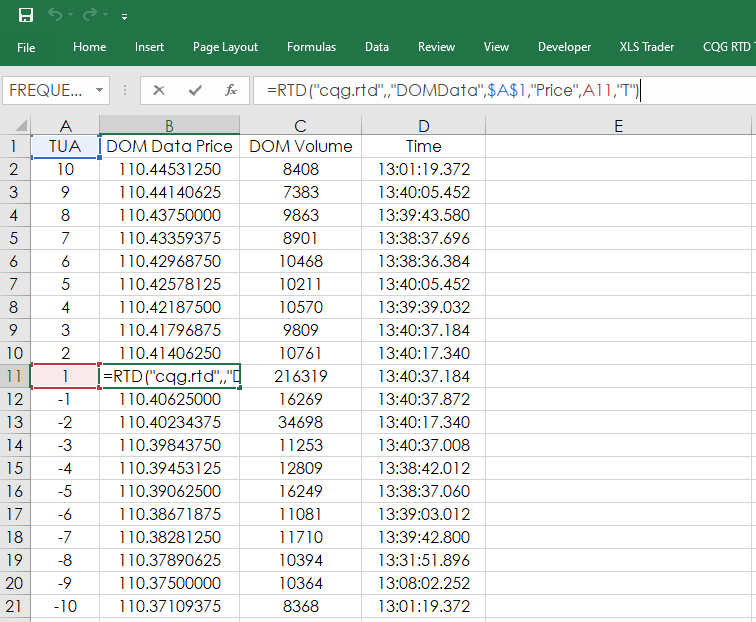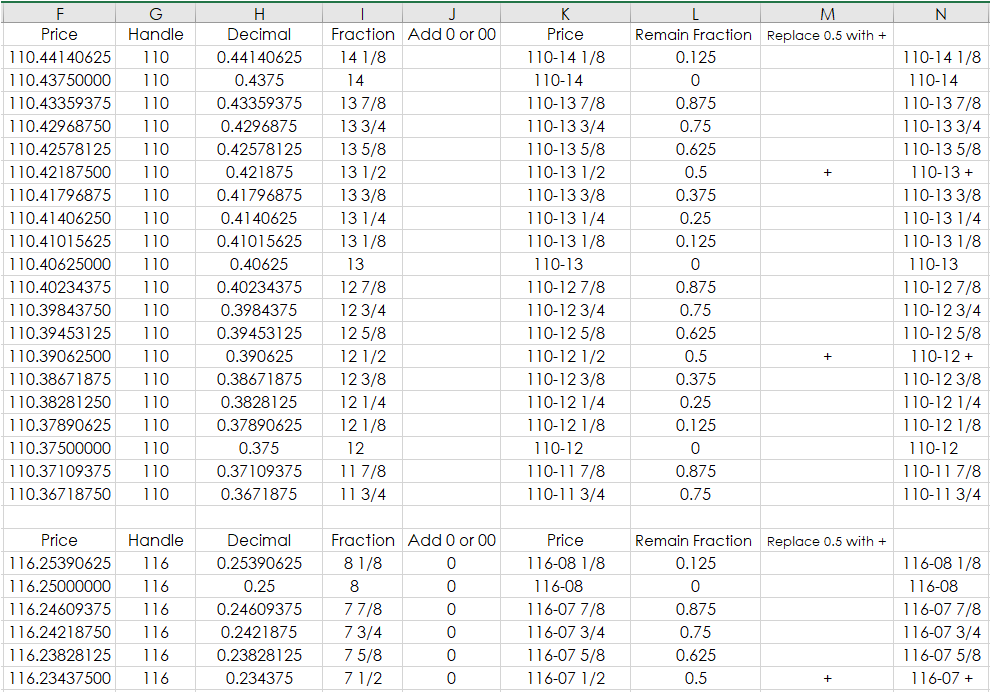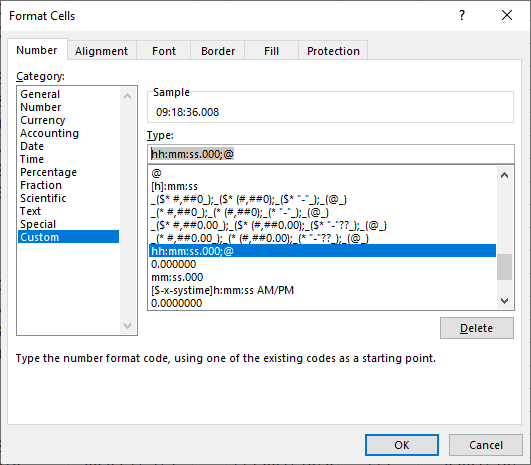# Displaying Fixed Income DOM Data in Excel

Fixed income traders may want to see fixed income futures prices for the book using a price format other than decimals or native. This article details the steps to converting decimal formatting to Solidus formatting (132.5078125 converted to 132-16’1/4).

CQG has five price formatting settings for fixed income futures markets using Contract Data Label RTD functions:

• Compact: For example 132162
• Decimal: For example 132.5078125
• Fractions: For example 132-16’2
• Fractions: For example 132’16.25
• Solidus: For example 132-16’1/4Below are four Excel RTD formulas using Contract Data labels to call for the day's last trade with data formats used (T, B, F, and D). The price output is shown at the end of the formula.

```=RTD("cqg.rtd",,"ContractData","TYA?","LastTradeToday",,"T") = 121.734375

```

Using "T" returns the price in a decimal format. Using "B" returns the value in 32nds and is formatted as a text cell. If using "F," you must format the Excel cell font with CQG Swiss font. You will see "+" and "^" for an uptick and the down arrow for a down tick. This cell is also set to text format. Finally, if you use "D," the default format (if you do not enter anything in the formula) will return the native number.

If you use “B” then Excel/RTD will format the price using the same settings from CQG (currently set to Solidus displayed in the image above):

`=RTD("cqg.rtd",,"ContractData","TYA?","LastTradeToday",,"B") = 132-14'+`

However, if you want to track the book of the bids and the offers for a fixed income futures market you can use this RTD Formula for the best Ask price:

```=RTD("cqg.rtd",,"DOMData",”TUA”,"Price",1,"T")
```

And, for the best Bid price:

`=RTD("cqg.rtd",,"DOMData",”TUA”,"Price",-1,"T")`

The 1 and the -1 above are the best Ask and best Bid respectively. The CBOT on Globex displays up to ten queues. To display the entire book the RTD calls would reference values from 1 to 10 and from -1 to -10.

Also, notice the use of “T” in the RTD formula and this calls a decimal value. The only other choice is “D”. “B” and “F” formatting is not available.Such as:

• 110.41015625 = 110-13 1/8
• 110.421875 = 110-13 +

One issue to note: This process requires the prices to be converted to text. One tab has the conversion processes for symbols TUA, Z3N, FVA, TYA, and USA and the Main Display tab uses the Solidus price formatting.On the Data tab column F is pulling in the prices using the decimal format from column B. Column G is pulling out the Note or Bond’s handle and converting it to text:

`=TEXT(TRUNC(F2),"###")`

Column H is separating the decimal price from the handle:

`=F2-G2`

Column I is converting the decimal price to a fraction of 32nds:

`=TEXT(H2*32,"# ?/?")`

Column J is dealing with the issue that if the Note price is between 1/32 and 10/32 then an additional zero (0) is needed in the price right after the separator. And if the price is less than 1/32nd then two 0s are required.

• 116.2421875 = 116-07 ¾
• 116.015625 = 116-00 +
`=IFERROR(IF((I2*1)<10,IF(I2*1<1,0,0),""),"00")`

Column K is merging the handle with the fraction and including “-“ as the separator.

`=G2&"-"&J2&I2`

Then column L is finding the decimal fraction of the 32nds.

`=MOD(H2*32,1)`

Column L is then reviewed by column M to replace “0.5” with the “+” label.

Finally, column N uses either column K’s prices or if the price does have “+” then the “+” is used instead of ½.

`=IF(M2="+",LEFT(K2,LEN(K2)-3)&M2,K2)`

Finally, on the main display tab the time of the DOM updates is shown. This time periods are in milliseconds. Excel does not have a format for displaying time in milliseconds, but you can create a custom time format and apply it to the time columns.

`hh:mm:ss.000;@`Requires CQG Integrated Client or QTrader. Strongly recommended: Microsoft Office Professional Excel 2016, 2019, 32 or 64-bits installed on your computer, not in the Cloud.